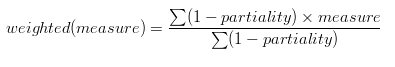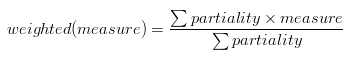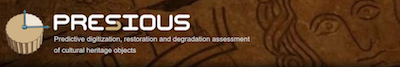### The Track

The problem of retrieving 3D shapes using queries with partial data is an open and challenging problem. Moreover, with the increasing use of cheap 3D devices such as scanners and RGB-D cameras in real-world applications, this problem is receiving special attention due to its increased potential for model creation, repair and retrieval tasks. In this track, we aim at evaluating algorithms for partial shape retrieval using a large set of queries composed by views. Our general idea is to simulate a partial scan of a 3D model by generating point clouds from a number of views of the model. For each view, a point cloud is extracted and a varying number of views with several degrees of partiality are used for retrieval.

We believe that our track represents a next step in the evaluation of partial retrieval algorithms compared to previous tracks. In addition, novel measures are introduced in order to give prominence to the level of “partiality” of each partial query. In this way, we want to reduce the bias introduced when comparing the queries with different level of difficulty.

### Organizers

• Ivan Sipiran - Department of Computer Science, University of Chile
• Rafael Meruane - Department of Computer Science, University of Chile
• Benjamin Bustos - Department of Computer Science, University of Chile
• Tobias Schreck - Department of Computer and Information Science, University of Konstanz

### The Target Set

We will use a subset of the SHREC'09 Generic Retrieval Benchmark. We selected 20 classes from that dataset with 18 models per class, so the number of models is 360. Model names begin with a T followed by the number of model, for instance T50.off, T240.off, and so on.

### The Query Set

For each model in the Target Set, we compute 20 partial views trying to cover the complete surface of the object. The process to obtain a view simulates the process of scanning, so the initial output of this step is a 3D point cloud giving a partial view of the model, as would be resulting from one static 3D scan (snapshot). Subsequently, a recontruction process computes the final set of 3D models (triangular surfaces) representing the partial views. In addition, we define the partiality of the view as the area ratio between the partial view and the entire object. Totally, there are 7200 queries.

### Ground-truth

The Target Set contains a ground-truth inherited from the SHREC'09 benchmark. For each query (a partial view), its relevance set is composed by the relevance set of the object which generates the view.

### The Evaluation Method

We use five measures to compute the effectiveness of algorithms:

• Mean Average Precision (MAP): Given a query, its average precision is the average of all precision values computed in each relevant object in the retrieved list. Given several queries, the mean average precision is the mean of average precision of each query.
• Nearest Neighbor (NN): Given a query, it is the precision at the first object of the retrieved list.
• First Tier (FT): Given a query, it is the precision when C objects have been retrieved, where C is the number of relevant objects to the query.
• Second Tier (ST): Given a query, it is the precision when 2*C objects have been retrieved, where C is the number of relevant objects to the query.
• Mean Query Rank (MQR): Given a query, the query rank is the position of the object which generates that query in the ranked list. Given several queries, the mean rank query is the mean of query ranks for each query.

We also use weighted measures regarding the partiality of the query set. For the precision-based measures (MAP, NN, FT, ST), we propose the following weighted version:For the rank-based measure (MQR), we propose the following weighted version:### Results submission

Participants submitted a matrix distance for each run. Up to 5 matrices may be submitted corresponding to different algorithms or a different parameter setting. The matrix distance must be stored in a file (with a white space as separator) and its size must be of 7200 x 360. The i-th row of the matrix corresponds with the distances from the i-th query (Q{i}.off) to every model in the target set. We will consider the same order imposed by the number in the name file for both target and query set. So entry A(i,j) corresponds to the distance from Q{i}.off to T{j}.off.

### Evaluation

The evaluation code and data can be downloaded here. If you use this dataset or the results, please cite the report as following:

• Sipiran I., Meruane, R., Bustos, B., Schreck, T., Li, B., Lu, Y., Johan, H.: SHREC'13 Track: Large-Scale Partial Shape Retrieval Using Simulated Range Images. Proc. Eurographics Worshop on 3D Object Retrieval (3DOR'13). Eurographics Association. 2013. [Bib]

### Supported by PRESIOUS

The work of Ivan Sipiran and Tobias Schreck was supported by EC FP7 STREP Project PRESIOUS, grant no. 600533. Benjamin Bustos has been partially funded by FONDECYT (Chile) Project 1140783.### PRISMA 3D scanner Dataset

You can also download and test our dataset generated with a 3D scanner and real objects.